# Multiplication principle + reason - math problems

#### Number of problems found: 69

• Hockey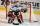Hockey match ended 8:2. How many different matches could be?
• DiceWe throw five times the dice. What is the probability that six fits exactly twice?
• Seven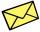Seven friends agree to send everyone a holiday card. How many postcards were sent?
• Tricolors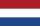From the colors - red, blue, green, black and white, create all possible tricolors.
• Two-element combinations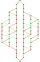Write all two-element combinations from elements a, b, c, d.
• Six on diceWhat is the probability that when throwing two dice will fall at least one six?
• Word MATEMATIKAHow many words can be created from the phrase MATEMATIKA by changing the letters' order, regardless of whether the words are meaningful?
• Chess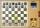How many different ways can initiate a game of chess (first pass)?
• One threeWe throw two dice. What is the probability that max one three falls?
• Bookshelf and books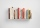How many ways can we place 7 books in a bookshelf?
• Seating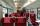How many ways can 6 people sit on 6 numbered chairs (e. g. , seat reservation on the train)?
• Three-digitHow many three-digit natural numbers do not have the number 7?
• Sum or productWhat is the probability that two dice fall will have the sum 7 or product 12?
• Hockey matchThe hockey match ended with result 3:1. How many different storylines may have the match?
• Gold, silver, bronzeHow many ways can we divide gold, silver, bronze medails if there are 6 people competing?
• Three digits numberHow many are three-digit integers such that in they no digit repeats?
• Toys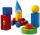3 children pulled 12 different toys from a box. Many ways can be divided toys so that each children had at least one toy?
• Twenty-fiveHow many three-digit natural numbers are divisible by 25?
• A jackpot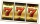How many times must I play this jackpot to win? A jackpot of seven games having (1 X 2), i. E. , home win or away win.
• Points in planeThe plane is given 12 points, 5 of which is located on a straight line. How many different lines could by draw from this points?

Do you have an interesting mathematical word problem that you can't solve it? Submit a math problem, and we can try to solve it.

We will send a solution to your e-mail address. Solved examples are also published here. Please enter the e-mail correctly and check whether you don't have a full mailbox.

Please do not submit problems from current active competitions such as Mathematical Olympiad, correspondence seminars etc...